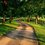# [Polynomials] Showing the Motzkin Polynomial is Non-Negative using the AM-GM Inequality

Let's take a look at the Motzkin polynomial $P(x,y) = x^4y^2+x^2y^4 +1-3x^2y^2.$ How can we show that this polynomial satisfies $P(x,y) \geq 0$ on $\mathbb{R^2}$?

We can look to the arithmetic mean-geometric mean (AM-GM) inequality to show this. The AM-GM inequality states that the arithmetic mean of a list of non-negative real numbers is greater than or equal to the geometric mean of the same list. Applying this inequality to the list of numbers $x^2y^4, x^4y^2, 1$, we can compute the arithmetic mean $\frac{x^2y^4 + x^4y^2 +1}{3},$ and the geometric mean $\sqrt{(x^2y^4)(x^4y^2)\cdot 1} = \sqrt{x^6y^6} = x^2y^2.$

Hence the inequality gives $\frac{x^2y^4 + x^4y^2 +1}{3} \geq x^2y^2$ Multiplying both sides by 3 and rearranging, $x^2y^4 + x^4y^2 +1 - 3x^2y^2 \geq 0$ as required.

We see that the AM-GM inequality provides a simple yet effective way to show whether polynomials over a real field are non-negative (or non-positive). You can also try showing that the polynomial $Q(x,y) = 2x^4+5y^4-x^2y^2+2x^3y$ over a real field, is non-negative as an exercise.Note by Bright Glow
3 years, 2 months ago

This discussion board is a place to discuss our Daily Challenges and the math and science related to those challenges. Explanations are more than just a solution — they should explain the steps and thinking strategies that you used to obtain the solution. Comments should further the discussion of math and science.

When posting on Brilliant:

• Use the emojis to react to an explanation, whether you're congratulating a job well done , or just really confused .
• Ask specific questions about the challenge or the steps in somebody's explanation. Well-posed questions can add a lot to the discussion, but posting "I don't understand!" doesn't help anyone.
• Try to contribute something new to the discussion, whether it is an extension, generalization or other idea related to the challenge.

MarkdownAppears as
*italics* or _italics_ italics
**bold** or __bold__ bold
- bulleted- list
• bulleted
• list
1. numbered2. list
1. numbered
2. list
Note: you must add a full line of space before and after lists for them to show up correctly
paragraph 1paragraph 2

paragraph 1

paragraph 2

[example link](https://brilliant.org)example link
> This is a quote
This is a quote
    # I indented these lines
# 4 spaces, and now they show
# up as a code block.

print "hello world"
# I indented these lines
# 4 spaces, and now they show
# up as a code block.

print "hello world"
MathAppears as
Remember to wrap math in $$ ... $$ or $ ... $ to ensure proper formatting.
2 \times 3 $2 \times 3$
2^{34} $2^{34}$
a_{i-1} $a_{i-1}$
\frac{2}{3} $\frac{2}{3}$
\sqrt{2} $\sqrt{2}$
\sum_{i=1}^3 $\sum_{i=1}^3$
\sin \theta $\sin \theta$
\boxed{123} $\boxed{123}$